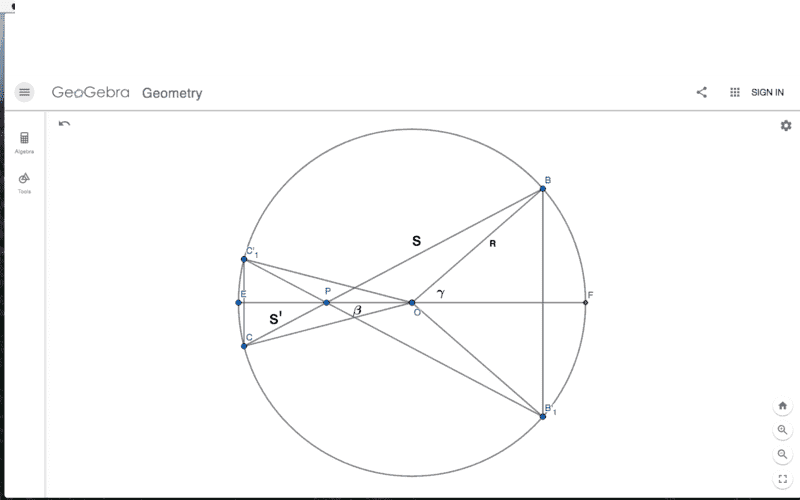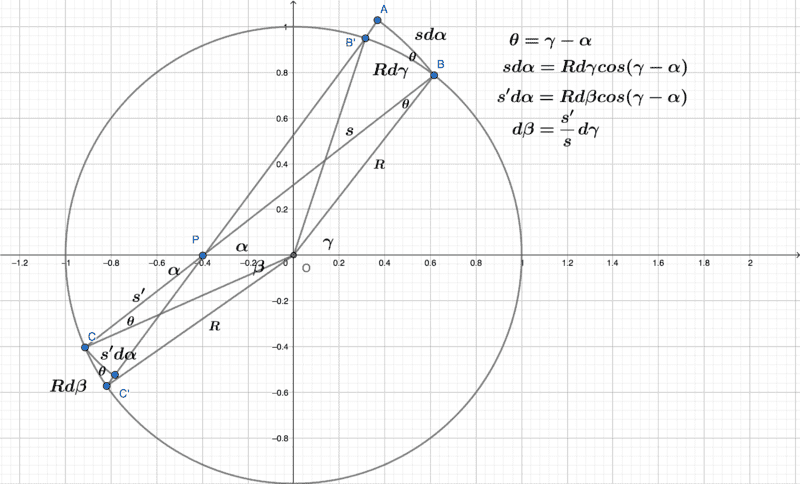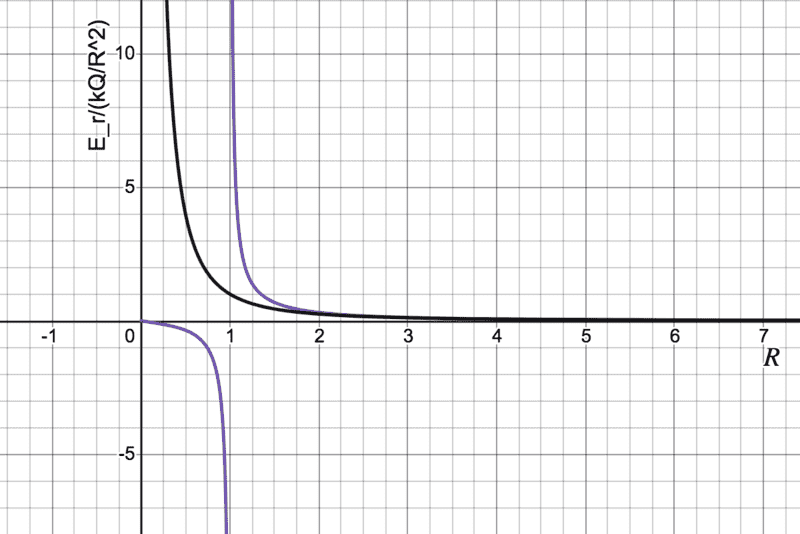# Electric field at a point within a charged circular ring

Delta2
Homework Helper
Gold Member
@vcsharp2003, check the video by @Steve4Physics at post #45, it has a more clever way of calculating ##\cos a## than using sine law.

P.S it seems that even with the video approach we end up with the same result as at post #21, the only difference is that we don't need the absolute value as I had noted. It is of these strange cases in math where you do two mistakes and you end up with the correct result ,lol.

Last edited:
•vcsharp2003
hutchphd
Homework Helper
I did not understand what you meant. Your definition of cylindrical symmetry is extraordinarilly restrictive: only infinitely long right cylinders are included. As mentioned above
Apart from one related to GR, all the items I could find online regard cylindrical, circular, azimuthal and axial symmetry as interchangeable terms.
yours is not the customary definition of cylindrical symmetry. You are welcome to use your own idiosyncratic definitions. You are not welcome to define the rest of the community as incorrect.
Good to clear this up.

•Delta2
Delta2
Homework Helper
Gold Member
yours is not the customary definition of cylindrical symmetry. You are welcome to use your own idiosyncratic definitions. You are not welcome to define the rest of the community as incorrect.
It might not be the customary definition, but in my opinion the rest of the community is incorrect and the fact that they use many different names for it (axial, azimuthal, cylindrical, circular) proves that the community doesn't know what they talk about.

Cylindrical symmetry is the symmetry the cylinder has, and it is symmetry both with respect to z and ##\phi## not only with ##\phi##. Period. The community is simply wrong on this. (Mad scientist talking :P).

•hutchphd
Here's a decent video (though a bit long at 13mins) deriving the field at a point inside a charged ring...

Thankyou for the excellent video.

•Steve4Physics
haruspex
Homework Helper
Gold Member
2020 Award
I did not understand what you meant. Your definition of cylindrical symmetry is extraordinarilly restrictive: only infinitely long right cylinders are included. As mentioned above

yours is not the customary definition of cylindrical symmetry. You are welcome to use your own idiosyncratic definitions. You are not welcome to define the rest of the community as incorrect.
Good to clear this up.
Not sure how to interpret "yours" in the above. You are addressing Delta2, yes? In the post of mine you quote, I was saying nearly all the online references I could find agree with you.

•hutchphd
Delta2
Homework Helper
Gold Member
Not sure how to interpret "yours" in the above. You are addressing Delta2, yes? In the post of mine you quote, I was saying nearly all the online references I could find agree with you.
Yes of course he is addressing me, he is just using you as his lawyer :P.

•hutchphd
haruspex
Homework Helper
Gold Member
2020 Award
Yes of course he is addressing me, he is just using you as his lawyer :P.
Research assistant?

•hutchphd and Delta2
hutchphd
Homework Helper
And I will always need all the help I can get.

bob012345
Gold MemberMy strategy in answering the original OP is to start with the sphere and show how the differential element of the field at point P exactly cancels for opposing differential rings such as the rings through points ##BB_1## and ##CC_1##. Then show for the 2D case of a charged ring they don't cancel thus avoiding messy integrations.

We know the integration for the whole sphere cancels at P but is the assertion that corresponding pairs of differential ring elements defined by the chords ##BC## and ##B_1C_1## also exactly cancel true?

Last edited:
haruspex
Homework Helper
Gold Member
2020 Award
Then show for the 2D case of a charged ring they don't cancel thus avoiding messy integrations.
That's a good approach, but it would not be quite enough to show that the same choice of pairing does not cancel; you would need to show that for this or some other pairing the net field is always the same way.

•bob012345
bob012345
Gold Member
That's a good approach, but it would not be quite enough to show that the same choice of pairing does not cancel; you would need to show that for this or some other pairing the net field is always the same way.
Thanks, so I take it that's a confirmation that pairings do cancel for the sphere. In either case, the pairings would always be general not based on specific angles.

haruspex
Homework Helper
Gold Member
2020 Award
Thanks, so I take it that's a confirmation that pairings do cancel for the sphere.
It must be the case that well chosen pairings cancel for the sphere, since we know the net field is zero. I have not checked whether this is true of the pairing you illustrate.
In either case, the pairings would always be general not based on specific angles.
Not sure what you mean by that. You would need a relationship between the angles, ##\phi=f(\theta)##, such that the band ##(\theta, \theta+\delta\theta)## cancels the band ##(f(\theta), f(\theta+\delta\theta))## .

•bob012345
bob012345
Gold Member
It must be the case that well chosen pairings cancel for the sphere, since we know the net field is zero. I have not checked whether this is true of the pairing you illustrate.
Not sure what you mean by that. You would need a relationship between the angles, ##\phi=f(\theta)##, such that the band ##(\theta, \theta+\delta\theta)## cancels the band ##(f(\theta), f(\theta+\delta\theta))## .The element from the right side is proportional to ##\Large \frac {R^2 sin(\gamma) d\gamma}{s^2}## and from the left side ##\Large \frac {R^2 sin(\beta) d\beta}{s'^2}## but in opposite directions along the axis. I know that ## sin(\beta) = {\Large \frac{s'}{s }}sin(\gamma)## and I believe ## d\beta = {\Large \frac{s'}{s }}d\gamma## making the two differential elements cancel at all angles. But I am having difficulty proving the relationship between ##d\beta## and ##d\gamma##. It is easy to see when the angles are very small and when ##s## and ##s'## are vertical but not easy to see in between. I did precision numerical calculations however to verify that the relation ## d\beta = {\Large \frac{s'}{s }}d\gamma## holds for wiggles around any angle. All that just makes ## \large \frac {R^2 sin(\beta) d\beta}{s'^2} → \large \frac {R^2 sin(\gamma) d\gamma}{s^2}## so the elements cancel.

Last edited:
haruspex
Homework Helper
Gold Member
2020 Award
View attachment 288165

The element from the right side is proportional to ##\Large \frac {R^2 sin(\gamma) d\gamma}{s^2}## and from the left side ##\Large \frac {R^2 sin(\beta) d\beta}{s'^2}## but in opposite directions along the axis. I know that ## sin(\beta) = {\Large \frac{s'}{s }}sin(\gamma)## and I believe ## d\beta = {\Large \frac{s'}{s }}d\gamma## making the two differential elements cancel at all angles. But I am having difficulty proving the relationship between ##d\beta## and ##d\gamma##. It is easy to see when the angles are very small and when ##s## and ##s'## are vertical but not easy to see in between. I did precision numerical calculations however to verify that the relation ## d\beta = {\Large \frac{s'}{s }}d\gamma## holds for wiggles around any angle. All that just makes

$$\large \frac {R^2 sin(\beta) d\beta}{s'^2} → \large \frac {R^2 sin(\gamma) d\gamma}{s^2}$$
Call angle BPO ##\alpha##.
Angle PBO= Angle PCO=##\gamma-\alpha##.
##s\delta\alpha=R\delta\gamma\cos(\gamma-\alpha)##
##s'\delta\alpha=R\delta\beta\cos(\gamma-\alpha)##.

•bob012345
bob012345
Gold Member
Considering the question of the charged ring in the OP, the paired differential elements are proportional to ##\Large \frac{R d\gamma}{s^2}## and ##\Large \frac{R d\beta}{s'^2}## and making use of ## d\beta = {\Large \frac{s'}{s }}d\gamma## gives ##\Large \frac{R d\gamma}{s^2}## and ##\Large \frac{R d\gamma}{ss'}## which do not cancel because ##s^2## is always larger than ##ss'## until the very end when they become equal as both become vertical in the plot. This is also where the integration ends. Also note even though ##s## and ##s'## depend on ##\gamma## and ##\beta## respectively, the product of ##ss'## is a constant ##ss' = (R+r)(R-r)## where ##r## is the distance point ##P## is from the center along the central axis of the circle. I ignored the cosine factors which make the integration messy because it is the same for both paired elements and the whole point of this approach is to answer the original question if the electric field is zero or not while avoiding doing the integration.

Last edited:
bob012345
Gold Member
Call angle BPO ##\alpha##.
Angle PBO= Angle PCO=##\gamma-\alpha##.
##s\delta\alpha=R\delta\gamma\cos(\gamma-\alpha)##
##s'\delta\alpha=R\delta\beta\cos(\gamma-\alpha)##.
Thanks. I finally understand your derivation for ##d\beta\ = \Large\frac{s'}{s} \large d\gamma##. The difference between ##B## and ##B'## and ##C## and ##C'## is greatly exaggerated.
.Last edited:
rude man
Homework Helper
Gold Member
Anyway, the answer is obviously no. Going close to the ring - what is the (simple) expression relating charge density to E field on the surface of a dielectric?

I
You could try writing down the integral for the net field at P and look for extrema as the distance of P from the centre of the hoop varies.
I think that would involve elliptical integrals which are best left alone here.

•bob012345
haruspex
Homework Helper
Gold Member
2020 Award
I think that would involve elliptical integrals which are best left alone here.
Since only extrema are of interest, it might not be necessary to solve the integral.

bob012345
Gold Member
Since only extrema are of interest, it might not be necessary to solve the integral.
Since the OP only asked if it is zero or not at one point along the radius, you would not have to actually do any integrals but just set the problem up. I found the formula worked out here*. The answer does involve Elliptical integrals of the first and second kind. If it wasn't enough to just look at the formula and see it is not zero, one can evaluate the elliptical integrals by lookup table at that point and see the value is not zero. I still think none of this is necessary based on the previous strategy.

$$E_r = \frac{2\pi R \lambda}{ 4πε_0} \frac{2}{ ^{\large \pi q^\frac{3 }{2}} (1−µ) }\frac{1 }{µ} (2R(1−µ) K(k)−(2R−µ(r +R))E(k))$$

where ##~~q = r^2 + R^2 +2rR ~~; k = \sqrt\mu## and ##\mu = \large \frac{4rR}{q}## and ##~~K(k) , E(k)## are the complete elliptical integrals of the first and second kind.

This can be simplified by using ##a = \large\frac{r}{R}## and noticing ##q = (R + r)^2 = R^2(1 + a)^2##

we get finally;

$$E_r = \frac{2 \lambda}{ 4πε_0 R} \left[\frac{1}{a} \left(\frac{K(k)}{(1+a)} - \frac{E(k)}{(1-a)}\right)\right],~~~a>0$$

or in the form with ##k_e =\large \frac{1}{4πε_0}## and ##Q = 2\pi R \lambda##;

$$E_r = \frac{ k_e Q}{ \pi R^2} \left[\frac{1}{a} \left(\frac{K(k)}{(1+a)} - \frac{E(k)}{(1-a)}\right)\right],~~~a>0$$

For ##a = \large \frac{2}{3}##, the ratio## \frac{ \Large E_r R^2}{\Large k_e Q}##gives ~-0.693 which is not zero.

since ##a =\Large \frac{r}{R}## and ##k = \Large \frac{2\sqrt a}{1+a}## as ##a → 0, E_r → 0## as ##a →1## ##E_r## diverges.

then as ##a, r →∞, k → 0, K(k), E(k) → \frac{\Large\pi}{2}## and ##E_r → \frac{ \large k_e Q}{ \ \Large r^2} ##

Here is a plot of what the field looks like. The black curve is the equivalent plot of a point charge equal to the ring for reference. The ##x## axis is distance of the point ##P## from the center of the ring in units of ##R##.*http://www.mare.ee/indrek/ephi/efield_ring_of_charge.pdf

Last edited:
•vcsharp2003